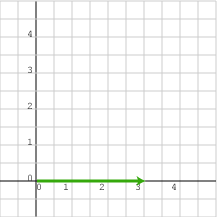created 08/26/97; revised 08/10/00; 07/22/03; 07/22/09; 01/10/15

# CHAPTER 4 — Vector Length

This chapter discusses the length of vectors and how length is computed using the column matrix representation of vectors. The next chapter will discuss another vector property, direction. Vectors of all dimensions have length and direction. But to make the discussion easier to visualize most of the examples in this chapter use vectors in 2D space.

• Length of 2D and 3D vectors
• Pythagorean formula
• Calculating the length of a vector from its column matrix representation
• Length of the zero vector
• Length of a negated vector

### QUESTION 1:What do you suppose is the length of the vector represented by:   (3, 0)T   ?